# SAS/ETS Examples

## Heteroscedastic Two-Stage Least Squares Regression with PROC MODEL

Contents | SAS Program

# Overview

Heteroscedastic two-stage least squares regression is a modification of the traditional two-stage least squares used to estimate simultaneous equation models when the disturbances are heteroscedastic. One can use the MODEL procedure in SAS/ETS to compute the two-stage heteroscedastic estimates.

# Details

Consider the general nonlinear simultaneous equations model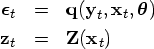whereis a real valued vector function of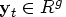,,, g is the number of equations, l is the number of exogenous variables, p is the number of parameters and t ranges from 1 to n.is a vector of instruments.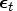is an unobservable disturbance vector with the following properties:The nonlinear two stage least squares (N2SLS) is a commonly used single equation estimation method that consists of using instrumental variables (IV) that are uncorrelated with the disturbances to obtain predicted values for the endogenous variables. Such predicted values replace the right hand side endogenous variables in the model to obtain consistent estimates of the parameters.

Formally, the N2SLS estimator is the value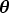that minimizes the quantity
A key assumption of N2SLS is that the disturbances are i.i.d.. In the presence of heteroscedasticity, the 2SLS estimator is still consistent but no longer efficient.

In such case, the nonlinear heteroscedastic 2SLS (NH2SLS) estimator is a single-equation estimator that attains a gain in efficency with respect to the N2SLS estimator by weighting observations with White's estimate of the error correlation matrix.

Formally, the NH2SLS estimator is obtained by minimizing the following quantity:
where
is a gk ×gk block-diagonal matrix with each block j given by
, for j = 1, ... ,g.

Note that the NH2SLS can be interpreted as a GMM estimator where the variance of the moment function V is taken to be block-diagonal. Each block, corresponding to each single equation, is estimated using the residuals from the N2SLS estimates.

The following table illustrates the relationship among some commonly used estimation methods for simultaneous equation models.

Table 1.1: Summary of PROC MODEL Estimation Methods
 Method Objective Function N2SLS
NH2SLS
N3SLS
GMM
where mn is first moment of the crossproduct
.

## Klein's Model

The following is the well known Klein's (1950) model I, along with an excerpt of the data. The complete data can be found in sas.htm , along with the code used in this example.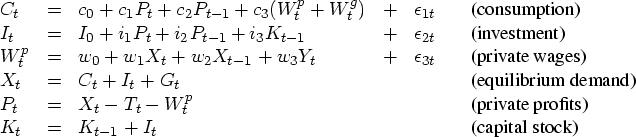options ls=80;
data klein;
input year c p w i x wp g t k wsum;

... more datalines ...

1934  48.7  12.3  30.6 -3.0  49.7  6.0   4.0   6.8  199.0  36.6
1935  51.3  14.0  33.2 -1.3  54.4  6.1   4.4   7.2  197.7  39.3
1936  57.7  17.6  36.8  2.1  62.7  7.4   2.9   8.3  199.8  44.2
1937  58.7  17.3  41.0  2.0  65.0  6.7   4.3   6.7  201.8  47.7
1938  57.5  15.3  38.2 -1.9  60.9  7.7   5.3   7.4  199.9  45.9
1939  61.6  19.0  41.6  1.3  69.5  7.8   6.6   8.9  201.2  49.4
1940  65.0  21.1  45.0  3.3  75.7  8.0   7.4   9.6  204.5  53.0
1941  69.7  23.5  53.3  4.9  88.4  8.5  13.8  11.6  209.4  61.8
;
run;


The endogenous variables are on the left hand side of the equations. The exogenous variables are government wage bills, Wg, government spending, G, indirect business taxes plus net export, T, the time trend in terms of years from 1931, Yt, and the constant terms.

The following PROC MODEL statements estimate the model using 2SLS and store the estimated variance of the moment functions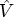in the dataset vdata via the OUTV= option.

   proc model data=klein;
instruments klag plag xlag wp g t yr;
c=c0 + c1*p + c2*plag + c3*wsum;
i=i0 + i1*p + i2*plag + i3*klag;
w=w0 + w1*x + w2*xlag + w3*yr;
fit c i w / 2sls outv=vdata vardef=n kernel=(bart,0,);
title '2sls results';
run;
quit;


The VARDEF=n and KERNEL=(bart, 0,) options have been used so that no correction for degrees of freedom or smoothing over time are performed when the matrix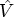is computed. See the chapter on the MODEL procedure in the SAS/ETS User's Guide for more details on these options.

One can estimate the model with H2SLS using the GMM method with the variance matrixset equal to a block diagonal matrix, where each block is given by the 2SLS estimate for the corresponding equation. See Greene (1997), p. 759 for details.

The following SAS statements create the block-diagonal matrix
using the matrix stored in the dataset vdata and print the first 15 observations.

   data vblkdiag;
set vdata;
if (eq_row ^= eq_col) then value=0;   /* make V block-diagonal */

proc print data=vblkdiag(obs=15);
title 'Block-diagonal V-matrix';
run;


The block-diagonal matrix
is then used in the VDATA= option of the MODEL procedure, so as to obtain the H2SLS estimates via GMM estimation. Note that all the exogenous variables are used as instruments. Also note that the NOGENGMMV option has been turned on in the FIT statement. This specifies the use of GMM variance under the optimal weighting matrix instead of the arbitrary weighting matrix. See the Estimation Methods in the SAS/ETS User's Guide.

   proc model data=klein;
instruments klag plag xlag wp g t yr;
c=c0 + c1*p + c2*plag + c3*wsum;
i=i0 + i1*p + i2*plag + i3*klag;
w=w0 + w1*x + w2*xlag + w3*yr;
fit c i w / no2sls gmm vdata=vblkdiag vardef=n nogengmmv;
title 'H2SLS results';
run;
quit;


The results of the estimation are shown in Figure 1. You can compare them with Greene (1997), p. 760.

 H2SLS results

The MODEL Procedure

Nonlinear GMM Parameter Estimates
Parameter Estimate Approx Std Err t Value Approx
Pr > |t|
c0 14.74433 1.1596 12.71 <.0001
c1 0.075792 0.0936 0.81 0.4291
c2 0.166269 0.0825 2.02 0.0599
c3 0.849365 0.0356 23.85 <.0001
i0 21.40696 6.3296 3.38 0.0035
i1 0.18586 0.1299 1.43 0.1706
i2 0.551308 0.1253 4.40 0.0004
i3 -0.16056 0.0305 -5.27 <.0001
w0 2.674615 0.7915 3.38 0.0036
w1 0.455802 0.0300 15.21 <.0001
w2 0.110765 0.0323 3.43 0.0032
w3 0.1306 0.0237 5.51 <.0001

Figure 1: H2SLS Results

# References

Greene, W.H. (1997), Econometric Analysis, Third Edition, Upple Saddle River, NJ: Prentice Hall, Inc.

Klein, L. (1950), Economic Fluctuations in the Unites States 1921-1940. New York: John Wileys and Sons.

SAS Institute, Inc. (1999), SAS/ETS User's guide, Version 8, Volume 2, Cary, NC: SAS Institute, Inc.

SAS Institute, Inc. (2003), SAS/ETS User's guide, Version 9, Cary, NC: SAS Institute, Inc.